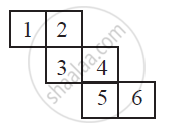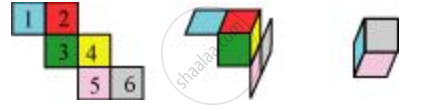Share

# Can this Be a Net for a Die? Explain You Answer - CBSE Class 7 - Mathematics

ConceptNets for Building 3-d Shapes

#### Question

Can this be a net for a die?#### Solution

The given net can be folded as follows.It can be observed that the opposite faces of the dice so formed have 2 and 5, 1 and 4, 3 and 6 on them. The sum of the numbers on the opposite faces comes to 7, 5, 9 respectively. However, in case of a dice, the sum of the numbers on the opposite faces should be 7. Hence, this net is not of a dice.

Is there an error in this question or solution?

#### APPEARS IN

NCERT Solution for Mathematics for Class 7 (2018 to Current)
Chapter 15: Visualising Solid Shapes
Ex. 15.10 | Q: 3 | Page no. 281
Solution Can this Be a Net for a Die? Explain You Answer Concept: Nets for Building 3-d Shapes.
S